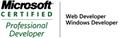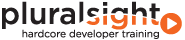Posts
208
1144
51
Interesting LINQ Exercise

A co-worker posed an interesting LINQ problem to me tonight so I figured I’d share.  They had a collection of items and wanted an algorithm that would create a “collection of collections” where the first three items would be grouped together, second three items, on so on.  For example, given a sequence like this: { “a”, “b”, “c”, “d”, “e”, “f”, “g”, “h” }, it would create a structure that contained 3 groups – the first element would be { “a”, “b”, “c” }, the second would be { “d”, “e”, “f” } and the third { “g”, “h” }. They already had an algorithm working fine but it was using a “brute force” approach and took about 15-20 lines of code.  It “felt like” you could solve the same problem more elegantly with a LINQ solution and with less lines of code.

Here was my first solution:

`   1:  var list = new List<string> { "a", "b", "c", "d", "e", "f", "g", "h" };`
`   2:  var results = new List<IEnumerable<string>>();`
`   3:  int numBlocks = list.Count % 3 == 0 ? list.Count / 3 : (list.Count / 3) + 1;`
`   4:   `
`   5:  for (int i = 0; i < numBlocks; i++)`
`   6:  {`
`   7:      results.Add(list.Skip(i * 3).Take(3));`
`   8:  }`

First, I have to figure out the number of “blocks” or elements that the structure (the collection of collection) should have.  Next, for each “block position” I leverage the LINQ Skip method in conjunction with the Take method (essentially like a paging operation).  I was reasonably happy with the first solution but I kept feeling like I could do it with less lines of code and without the “for” loop.  The problem with eliminating the for loop is that it was providing the outer loop and with LINQ you basically have to loop over something.  After a little digging, I came across the Enumerable Range method which I hadn’t used before.  This allowed me to solve the problem with 1 line of code:

`   1:  var list = new List<string> { "a", "b", "c", "d", "e", "f", "g", "h" };`
`   2:  int numBlocks = list.Count % 3 == 0 ? list.Count / 3 : (list.Count / 3) + 1;`
`   3:  var results = Enumerable.Range(0, numBlocks).Select(i => list.Skip(i * 3).Take(3)).ToList();`

Now we’ve got our collection of collection and can iterate over it:

`   1:  foreach (var item in results)`
`   2:  {`
`   3:      foreach (var innerItem in item)`
`   4:      {`
`   5:          Console.WriteLine(innerItem);`
`   6:      }`
`   7:  }`

While I was originally happy that I found a way to do it with 1 line of code, it’s not really a great solution because it’s just a little “too clever”.  The for loop is going to be easier to understand for the poor developer that comes behind you and has to maintain your code.  Also, the second solution is not as efficient because the Range method creates an array just so i can loop over it just so it can make it workable in LINQ.  Bottom line, the first solution is better.  But of course, if someone has an even better solution, please do not hesitate to share it in the comment section below.

To take it one step further, the LINQ SelectMany method will allow you to take the collection and collections and flatten it back out to the original single collection like this:

`   1:  var flattened = results.SelectMany(x => x);`
posted on Thursday, August 6, 2009 4:24 PM Print# re: Interesting LINQ Exercise
Rik Hemsley
8/9/2009 8:43 PM
Perhaps this sort of thing?

public static IEnumerable<IEnumerable<T>> Group<T>(this IEnumerable<T> enumerable, Func<T, long, bool> groupTrigger)
{
long index = 0;
var group = new List<T>();

foreach (var item in enumerable)
{

if (groupTrigger(item, index))
{
yield return group;
group = new List<T>();
}

++index;
}

if (group.Any())
yield return group;
}# re: Interesting LINQ Exercise
Jose Silva
8/9/2009 9:48 PM
My only advice is always keep your code simple and readable. This is the smart way to go. Too much refactoring can harm you (or someone else) in the future. And also remember, what you know today you might not remember tomorrow...

So keep it simple and straightforward (my own KISs). Don't be a cowboy developer :)# re: Interesting LINQ Exercise
Chris Fairles
8/12/2009 2:26 AM
I use something like:

public static IEnumerable<IEnumerable<T>> Group<T>(this IEnumerable<T> source, int blockSize)
{
int numBlocks = source.Count() % blockSize == 0 ? source.Count() / blockSize : (source.Count() / blockSize) + 1;
for (int i = 0; i < numBlocks; i++)
{
yield return source.Skip(i * blockSize).Take(blockSize);
}
}

int [] i = new int[] { 1,2,3,4,5,6,7,8 };

foreach(var groupOfThree in i.Group(3))
{
// groupOfThree == {1,2,3} then {4,5,6} then {7,8}
}# re: Interesting LINQ Exercise
Vince Panuccio
4/26/2010 4:39 AM
I came up with this solution

string[] ss = new string[] {"a","b", "c", "d", "e", "f", "g", "h"};

int start = 0;

var results =
from s in ss.Where ((s,i) => i % 3 != 0)
let grpOfthree = ss.Skip(start).Take(3)
let newStart = (start+=3)
select new {
Grp=grpOfthree
};# re: Interesting LINQ Exercise
Vince Panuccio
4/26/2010 4:42 AM
Oops, here is a correction :-)

string[] ss = new string[] {"a","b", "c", "d", "e", "f", "g", "h"};

int start = 0;

var results =
from s in ss.Where ((s,i) => { return i % 3 != 0 && i > start;})
let grpOfthree = ss.Skip(start).Take(3)
let newStart = (start+=3)
select new {
Grp=grpOfthree
};

results.Dump();# re: Interesting LINQ Exercise
Joost Morsink
6/4/2010 6:25 AM
Because using Skip and Take repeatedly for every group, creating a O(N^2) algorithm, I suggest using the indexed select method and a group by for a O(N) algorithm:

var lst = new List<string> { "a", "b", "c", "d", "e", "f", "g", "h" };
var q = lst.Select((x, idx) => new { x, idx }).GroupBy(y => y.idx/3, y => y.x);# re: Interesting LINQ Exercise
Toon
9/1/2011 8:49 AM
Hey Joost Morsink!

That looks really cool! Thanx a lot!# my own solution to this problem
12/24/2011 9:19 AM
Hi, I came up with my own solution to this problem. I'm just getting started with LINQ so I'm not sure if this the best way to do things.

List<string> letters = new List<string> { "a", "b", "c", "d", "e", "f", "g", "h" };
IEnumerable<IEnumerable<string>> lettersQuery = from letter in letters
group letter by letters.IndexOf(letter) / 3;
foreach(var letter in lettersQuery){
foreach (var member in letter)
{
Debug.Write(member+" ");
}
Debug.Write("\n");
}

In a nutshell my query creates groups of elements based on their index value within the original List. This works because integer division by 3 yields the same value for every group of three items( 3/3 = 4/3 = 5/3 ).# re: Interesting LINQ Exercise
Brian
10/23/2013 5:03 PM
Joost probably has the best solution to the problem. My first response was the following ,which is similar to Joost's solution, although it does not handle the case of data.Count % 3 == 1 in the same fashion (instead of the final group containing one element, the last two groups each contain two).

List<...> data = ...;
var query = data.Select((d, i) => new { dat = d, idx = i })
.GroupBy(o => o.idx % Math.Ceiling((double)data.Count / 3), o => o.dat);

foreach (var group in query)
{
Console.Write({0}: [", group.Key); // group.Key is a number in the [0..n] range, where n+1 is the number of groups
foreach (var item in group)
{
Console.Write("{0},", item);
}
Console.WriteLine("{0}]", (char)8); // Backspace character, deleting extra comma
}# re: Interesting LINQ Exercise
Michelle
2/22/2015 9:58 AM
Personally I would choose the simplest and most readable solution which is:

var list = new List<string> { "a", "b", "c", "d", "e", "f", "g", "h" };
var results = Enumerable.Range(0, list.Count).GroupBy(i => i/3);

### Post Comment

Title *
Name *
Email
Comment *
Verification